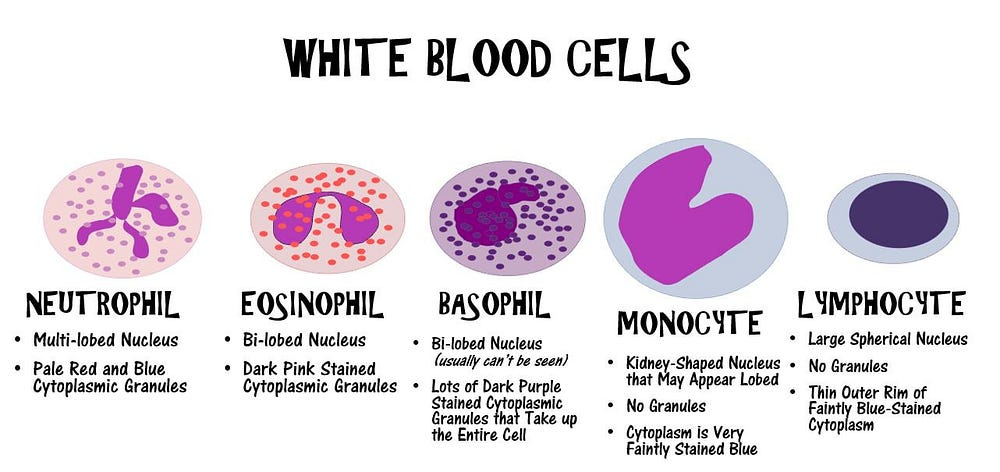# Week 5: Blood Cell Classification

Source: Deep Learning on Medium# CNN architecture used in Related Work

The architecture consists of 7 layers, the first 5 layers feature extraction, the last 2 layers fully connected.

In the first convolutional layer, kernelsize = 5, stride = 1, padding = 0, number of filters = 16. Then Maxpooling is applied.Kernel = 2, stride = 2

In the second convolutional layer, kernelsize = 3, stride = 1, padding = 0, number of filters = 32. Then Maxpooling is applied.Kernel = 2, stride = 2

In the third convolutional layer, kernelsize = 3, stride = 1, padding = 0, number of filters = 64.

ReLu was used as activation function in each convolutional layer.

Finally, Softmax is used as the classifier in the fully connected layer.

The number of class in related work class but the number of classes in our project 4 and in order to use this model, we resized the pictures to 100×100 format.